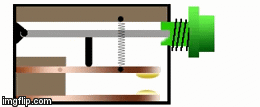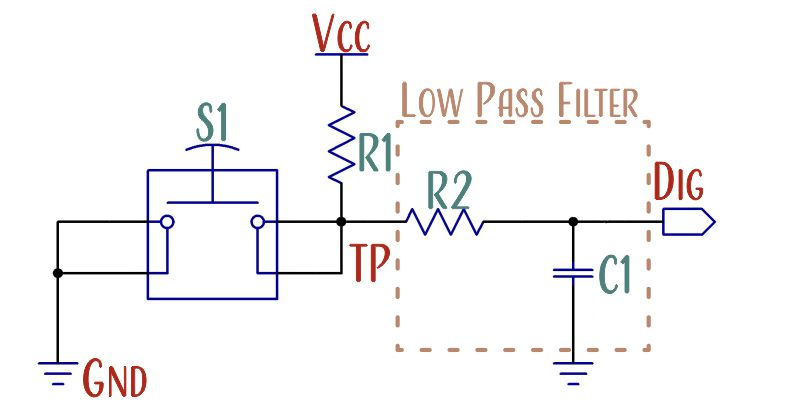## Circuit Analysis

Before you layout a circuit, it's generally a good idea to understand what is happening in your schematic.  And before you lay out a schematic, it's generally a good idea to understand what is happening in your block diagram."This book is a bit technical.  If you are a beginner, it's okay that you don't understand everything that follows -- your first project will still be successful.  Try to pick up as much as you can, and ask questions in the forums!"

### 7. Input Switches

We often think of switches as simple devices that exist in one of two states: on or off, open or closed.  And in the steady-state, long after a transition, that is how they behave. But during the transition between states, many switches tend to misbehave as their contact points make and break contact several or even dozens of times.##### This image shows mechanical bouncing.  Image origin unknown.

It has to do with the way switches are designed.  Many switches are designed with springs, compliant mechanisms, or "snap-action" mechanisms that mechanically hold the switch element in place on contacts  Like a ball bouncing on the ground, the contact elements often bounce immediately after the transition. So instead of a single transition between states, there can be dozens of transitions back and forth and back again.##### This rising-edge contact bounce from Maxim Integrated shows around 2-dozen logic transitions.

Each transition can cause electromagnetic noise and trigger the input on an integrated circuit.  Imagine the switch above used in a counting application. Each switch press might be seen as 10 counts by an integrated circuit.

## Switch Debouncing

We're electrical engineers, not mechanical engineers.  So we have no control over the switch design, and cannot control the bounce.  We just have to deal with it. Our two options are to design an electric circuit to deal with the bounce or write software code to deal with the bounce.  Since this course focuses on electrical design rather than software design, we chose a simple hardware debounce circuit. Datasheets sometimes recommend different debounce circuits or no debounce at all, but otherwise, this configuration is relatively standard.

####### One of the debounce circuits from the schematic.  In this circuit, a pullup resistor (R1) ties one side of the switch to the 3.3 V power rail and a low-pass filter connects the switch to the microcontroller and a 0.1 μF capacitor.

The circuit starts out with two resistors and one capacitor of unknown values.  R1 is simply a pull-up resistor while R2 and C1 form a low-pass filter. How did we select the values for R1, R2, and C1?  Start by separating the pull-up resistor from the low-pass filter.

The next section explains the calculations for the circuit.

# Circuit Analysis

When a potential difference exists across a resistor, charges move until the potential difference across the resistor is zero.  If there is no exit path for the charges, each unit of charge that crosses the resistor decreases the potential difference across the resistor ever so slightly, which then reduces the rate of charge flow.  This behavior is what gives RC circuits their characteristic exponential curvature.

## RC-Filter

When Switch S1 is closed, R2 is connected directly to ground and R1 is simply generating heat, in that instance, C1 and R2 are the only components of interest in the RC-Filter.  When S1 is open, R2 is connected to Vcc through R1, so R1, R2 and C1 must be included in the filter calculations.

### Capacitor Selection for RC-Filter

We can pick from a large array of capacitor values.  We need something with low equivalent series inductance (ESL) and low equivalent series resistance (ESR) and we need something that is inexpensive.  Those conditions all point towards small multilayer ceramic capacitors (MLCC). Since we're using 0.1 μF ceramics in dozens of spots in the circuit, we might as well use one here.  At this point, the choice is arbitrary, not calculated.

The switch datasheet states the settling time is 5 ms (0.005 s). If it was any greater, like the 10-20 ms seen in a relay, we might consider a larger-value capacitor in the 1 µF range.

Our goal is to design an RC circuit that transitions between Vcc and Logic Low in 5ms or greater.

### Resistor Selection for RC-Filter During Discharge

$\triangle t = 5\times10^{−3}\;\text{s} \hspace{10mm} C=0.1\times10^{−6}\;\text{F}$

$Vcc=3.3\;\text{V} \hspace{10mm} V_{\text{Logic Low Threshold}} = 1\;V$

$R_2=?$

$V_{\text{Logic Low Threshold}}=V_{\text{cc}}e^\frac{−\triangle t}{RC}\hspace{10mm}R_2=\frac{−\triangle t}{C\cdot ln(\frac{V_{\text{Logic Low Threshold}}}{V_{\text{cc}}})}$

$R_2=\frac{−5\times10^{−3}\;\text{s}}{0.1\times10^{−6}\;\text{F}\cdot ln(\frac{1\;V}{3.3\;V})}=41.9 k\Omega$

And we’d pick a resistor of similar value from the E24-series, or 43 kΩ, with the large tolerances of the capacitors and resistors, it doesn’t make much sense to select a resistor more precise than this.

### Pull-Up Resistor

The job of a pull-up resistor is to ensure a digital input is in a defined logic-state when no other circuit elements are acting on the input.  When the switch opens, the digital input “Dig” is connected to Vcc through the resistors R1 and R2, so they must both be included in the calculation.

$Vcc=3.3\;\text{V} \hspace{5mm} V_{\text{Logic High Threshold}} = 2\;\text{V} \hspace{5mm} R_2=41.9\;k\Omega$

$R_1=?$

$V_{\text{Logic High Threshold}}=V_{\text{cc}}\left ( 1− e^\frac{−\triangle t}{(R_1+R_2)\cdot C}\right )$

$R_1=\frac{−\triangle t}{C\cdot ln(1−\frac{V_{\text{Logic High Threshold}}}{V_{\text{cc}}})}−R_2$

$R_1=\frac{−5\times10^{−3}\;\text{s}}{0.1\times10^{−6}\;\text{F}\cdot ln(1−\frac{2\;V}{3.3\;V})}−41.9\;k\Omega=12\;k\Omega$

#### Lower Value Limit Caution

It’s not even close to a problem here, but if higher voltages are in play, a switch has a very low settling time, and a designer chooses a high value capacitance, it’s possible to have high currents flowing through the pull-up resistor.

To choose the lowest allowable value for a resistor, circuit designers should look at the condition where the switch is closed and the net labeled TP is shorted to ground.  The current will flow from Vcc to Gnd nets through R1. A very low-value resistor will allow a great deal of current to flow across it. The limits are placed by the energy dissipation of the resistor and the current available from the power supply.  Many of these tiny resistors have power dissipation factors of around 1/10 W. Using Ohm's Law and the Power Law, we can find the resistance and current requirements quickly.

$P=\frac{V^2}{R}$

$R=\frac{V^2}{P}$

$R=\frac{(3.3\;\text{V})^2}{0.1\;\text{W}}=110 \Omega$

$I=\frac{\triangle V}{R}$

$I=\frac{3.3\; V}{110\;\Omega}=30\;\text{mA}$

30 mA can easily exceed the recommended power output of some small button-cell batteries. So we'd definitely want a resistor with a value greater than 110 Ω.  Also keep in mind that multiple switches might be depressed at once, causing a large drain on a tiny power supply. In that case, you should decrease the capacitance and increase the resistance to reduce the current through the pull-up resistor.

Each transition can cause electromagnetic noise and trigger the input on an integrated circuit.  Imagine the switch above used in a counting application.  Each switch press might be seen as 10 counts by an integrated circuit."I think the last time I performed these calculations I was an undergraduate in college some 20 years ago.  Most of the time I look for an application example in the datasheet and Ctrl-C Ctrl-V the values into my schematic.""Do engineers even use external hardware debouncing anymore?  I think the trend over the last several years has been towards hardware debouncing inside the microcontroller, or software debouncing."